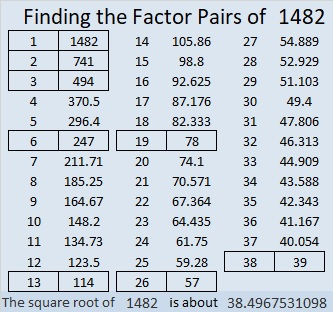# 1482 and Level 4

Contents

### Today’s Puzzle:

Can you write all the numbers from 1 to 10 in both the first column and the top row so that those numbers become the factors of the given clues?### Factors of 1482:

• 1482 is a composite number.
• Prime factorization: 1482 = 2 × 3 × 13 × 19
• 1482 has no exponents greater than 1 in its prime factorization, so √1482 cannot be simplified.
• The exponents in the prime factorization are 1, 1, 1, and 1. Adding one to each exponent and multiplying we get (1 + 1)(1 + 1)(1 + 1)(1 + 1) = 2 × 2 × 2 × 2 = 16. Therefore 1482 has exactly 16 factors.
• The factors of 1482 are outlined with their factor pair partners in the graphic below.### Another Fact about the Number 1482:

1482 is the hypotenuse of a Pythagorean triple:
570-1368-1482 which is (5-12-13) times 114.

This site uses Akismet to reduce spam. Learn how your comment data is processed.